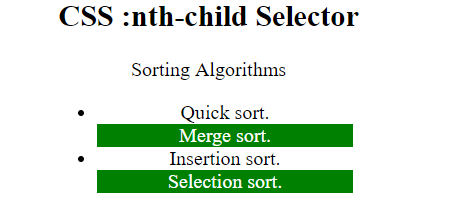Related Articles

# CSS | :nth-child() Selector

• Last Updated : 07 Jan, 2019

The :nth-child() selector in CSS is used to match the elements based on their position in a group of siblings. It matches every element that is the nth child.

Syntax:

```:nth-child(number) {
//CSS Property
}```

Where number is the argument that represents the pattern for matching elements. It can be odd, even or in a functional notation.

• odd: It represents elements whose position is odd in a series: 1, 3, 5, etc.
• even: It represents the elements whose position is even in a series: 2, 4, 6, etc.
• functional notation (<An+B>): It represents elements whose position of siblings matches the pattern An+B, for every positive integer or zero value of n.

Example-1: In this example every odd paragraph is selected. Formula used is 2n+1 i.e 1, 3, 5, etc paragraphs are selected.

 ```<``html``>``    ``<``head``>``        ``<``title``>CSS :nth-child Selector``        ``<``style``> ``            ``p:nth-child(2n+1) {``              ``background: green;``              ``color: white;``            ``}``        ````    ````    ``<``body` `style` `= ``"text-align:center"``>``        ``<``h1` `style` `= ``"color:green;"``>``            ``GeeksforGeeks``        ````        ``<``h2``>``            ``CSS :nth-child Selector``        ````     ` `        ``<``p``>A computer science portal for geeks.``        ``<``p``>Geeks classes an extensive classroom programme.``         ` `    `````

Output:Example-2: In this example every even <li> is selected i.e. 2, 4, 6, etc.

 ```<``html``>``    ``<``head``>``        ``<``title``>CSS :nth-child Selector``        ``<``style``> ``        ``li {``            ``width: 30%;``        ``}``            ``li:nth-child(even) {``              ``background: green;``              ``color: white;``            ``}``        ````    ````    ``<``body` `style` `= ``"text-align:center"``>``        ``<``h2``>``            ``CSS :nth-child Selector``        ````        ``<``p``>Sorting Algorithms``        ``<``ul``>``        ``<``li``>Quick sort.``        ``<``li``>Merge sort.``        ``<``li``>Insertion sort.``        ``<``li``>Selection sort.``         ` `        ````         ` `    `````

Output:Supported Browsers: The browser supported by :nth-child() selector are listed below:

• Apple Safari 3.2